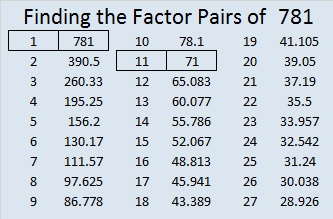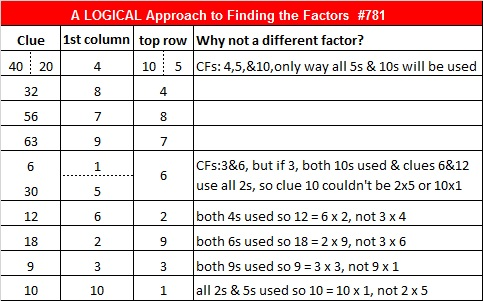# 781 and Level 6

• 781 is a composite number.
• Prime factorization: 781 = 11 x 71
• The exponents in the prime factorization are 1 and 1. Adding one to each and multiplying we get (1 + 1)(1 + 1) = 2 x 2 = 4. Therefore 781 has exactly 4 factors.
• Factors of 781: 1, 11, 71, 781
• Factor pairs: 781 = 1 x 781 or 11 x 71
• 781 has no square factors that allow its square root to be simplified. √781 ≈ 27.94637722.Can you solve today’s puzzle?Print the puzzles or type the solution on this excel file: 10-factors-2016

—————————————

Here’s more about the number 781:

781 is the sum of the 19 prime numbers from 7 to 79.

Thus 7 + 11 + 13 + 17 + 19 + 23 + 29 + 31 + 37 + 41 + 43 + 47 + 53 + 59 + 61 + 67 + 71 + 73 + 79 = 781

781 is a repdigit in base 5 and a palindrome in three other bases:

• 11111 BASE 5. Note that 625 + 125 + 25 + 5 + 1 = 781.
• 232 BASE 19. Note that 2(19²) + 3(19) + 2(1) = 781
• 1J1 BASE 20, J = 19 base 10. Note that 1(20²) + 19(20) + 1(1) = 781
• 141 BASE 26. Note that 1(26²) + 4(26) + 1(1) = 781

781 is also the sum of three squares five ways

• 27² + 6² + 4² = 781
• 24² + 14² + 3² = 781
• 24² + 13² + 6² = 781
• 21² + 18² + 4² = 781
• 21² + 14² + 12² = 781

—————————————This site uses Akismet to reduce spam. Learn how your comment data is processed.